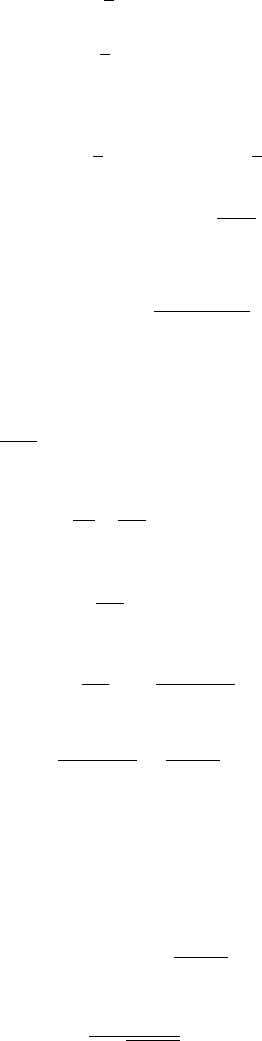# MAT244H1 Study Guide - Midterm Guide: Jordan Bell, Wronskian, Stationary Point

31 views5 pagesMat 244 Practice Test 3
Jordan Bell
July 25, 2013
You should be able to do all of the questions in this test given enough time.
This is certainly longer than the actual test will be. I wanted to give you more
worked out examples for calculus of variations.
1. Find the extremals of the functional
Zx2
x1
y2+y022ysin xdx.
Solution. f=y2+y022ysin x. So
f
y = 2y2 sin x, f
y0= 2y0.
Hence the Euler-Lagrange equation is
2y2 sin x= 2y00
i.e.
y00 y=sin x.
This is a second order ODE. The solution of the homogeneous equation is
c1ex+c2ex.
Now we use variation of parameters. y1=ex, y2=ex. The Wronskian is
W=2. g=sin x.
u1=Zgy2
Wdx =1
2Zsin xexdx
u2=Zgy1
Wdx =1
2Zsin xexdx
To compute u1, u2we need to use integration by parts. I won’t write this out;
it is something that you should be able to skillfully do if you have suﬃcient time,
and you should have absolutely no problem remembering how to do integration
by parts. You don’t have to do it in your head; unless it’s obvious to me, I
manually set uand dv and write out the integration by parts formula.
1
Unlock document

This preview shows pages 1-2 of the document.
Unlock all 5 pages and 3 million more documents.u1=1
4ex(cos x+ sin x)
u2=1
4ex(cos x+ sin x)
So the extremals of the functional Rx2
x1y2+y022ysin xdx are
y=c1ex+c2ex+1
4(cos x+ sin x) + 1
4(cos x+ sin x)
=c1ex+c2ex+sin x
2.
2. Find the extremals of the functional
I(y) = Zx2
x1
1+(y(x))2
(y0(x))2dx
This takes longer than I would want for a test question, but if I gave you
enough time I would expect you to be able to do something like this level of
diﬃculty.
Solution. f=1+y2
y02. Since fdoesn’t depend on x, the Euler-Lagrange
equation for this functional is
d
dx f
y0y0f= 0
which can also be written f
y0y0f=c1.
Now,
f
y0=2(1 + y2)
y03.
Hence 2(1 + y2)
y02+1 + y2
y02=c1
so
2+2y2+1+y2=c1y02
so
1 + y2=c1y02
so (in this step I change c1, which is logically acceptable untl we have introduced
c2)
y0=c1p1 + y2
i.e. dy
c1p1 + y2=dx.
2
Unlock document

This preview shows pages 1-2 of the document.
Unlock all 5 pages and 3 million more documents.